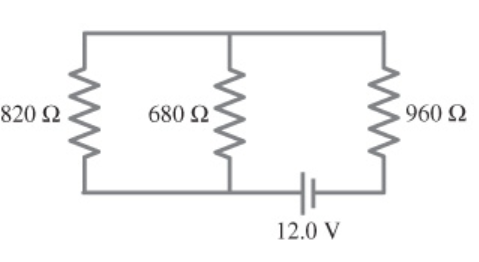# Problem: (a) Determine the equivalent resistance of the circuit shown in the figure. Express your answer to three significant figures and include the appropriate units.(b) Determine the voltage across 820 Ω resistor. Express your answer to two significant figures and include the appropriate units.(c) Determine the voltage across 960 Ω resistor. Express your answer to two significant figures and include the appropriate units.(d) Determine the voltage across 680 Ω resistor. Express your answer to two significant figures and include the appropriate units.￼

###### FREE Expert Solution

Equivalent resistance for series resistors:

$\overline{){{\mathbf{R}}}_{\mathbf{e}\mathbf{q}}{\mathbf{=}}{{\mathbf{R}}}_{{\mathbf{1}}}{\mathbf{+}}{{\mathbf{R}}}_{{\mathbf{2}}}}$

Equivalent resistance for parallel resistors:

$\overline{)\frac{\mathbf{1}}{{\mathbf{R}}_{\mathbf{eq}}}{\mathbf{=}}\frac{\mathbf{1}}{{\mathbf{R}}_{\mathbf{1}}}{\mathbf{+}}\frac{\mathbf{1}}{{\mathbf{R}}_{\mathbf{2}}}}$

(a) The 820Ω and the 680Ω resistor are in parallel.

R820,680 = (1/820 + 1/680)-1 = 371.7 Ω

83% (162 ratings)###### Problem Details

(a) Determine the equivalent resistance of the circuit shown in the figure. Express your answer to three significant figures and include the appropriate units.
(b) Determine the voltage across 820 Ω resistor. Express your answer to two significant figures and include the appropriate units.
(c) Determine the voltage across 960 Ω resistor. Express your answer to two significant figures and include the appropriate units.
(d) Determine the voltage across 680 Ω resistor. Express your answer to two significant figures and include the appropriate units.Frequently Asked Questions

What scientific concept do you need to know in order to solve this problem?

Our tutors have indicated that to solve this problem you will need to apply the Combining Resistors in Series & Parallel concept. You can view video lessons to learn Combining Resistors in Series & Parallel. Or if you need more Combining Resistors in Series & Parallel practice, you can also practice Combining Resistors in Series & Parallel practice problems.

What professor is this problem relevant for?

Based on our data, we think this problem is relevant for Professor Stolbov's class at UCF.